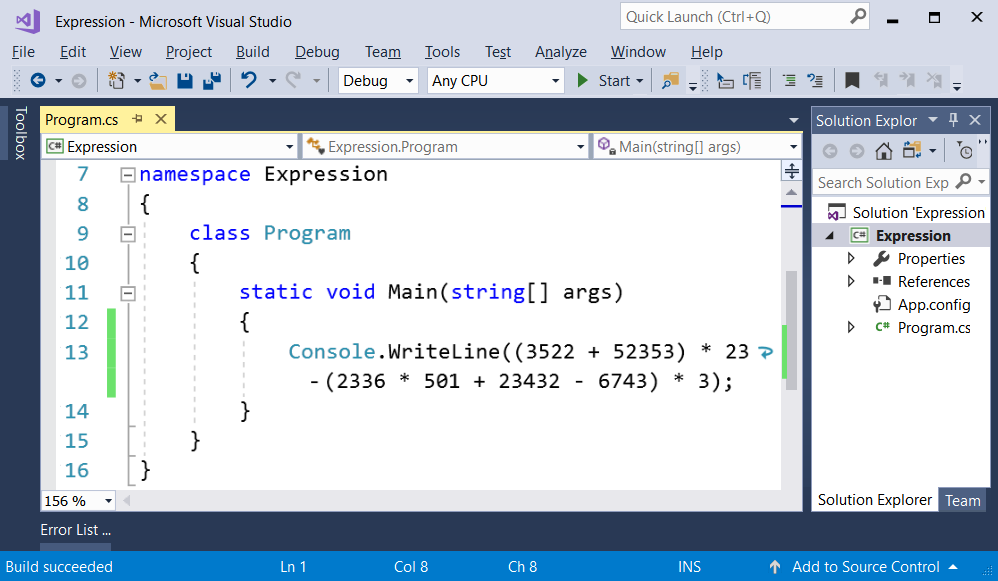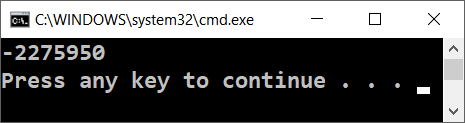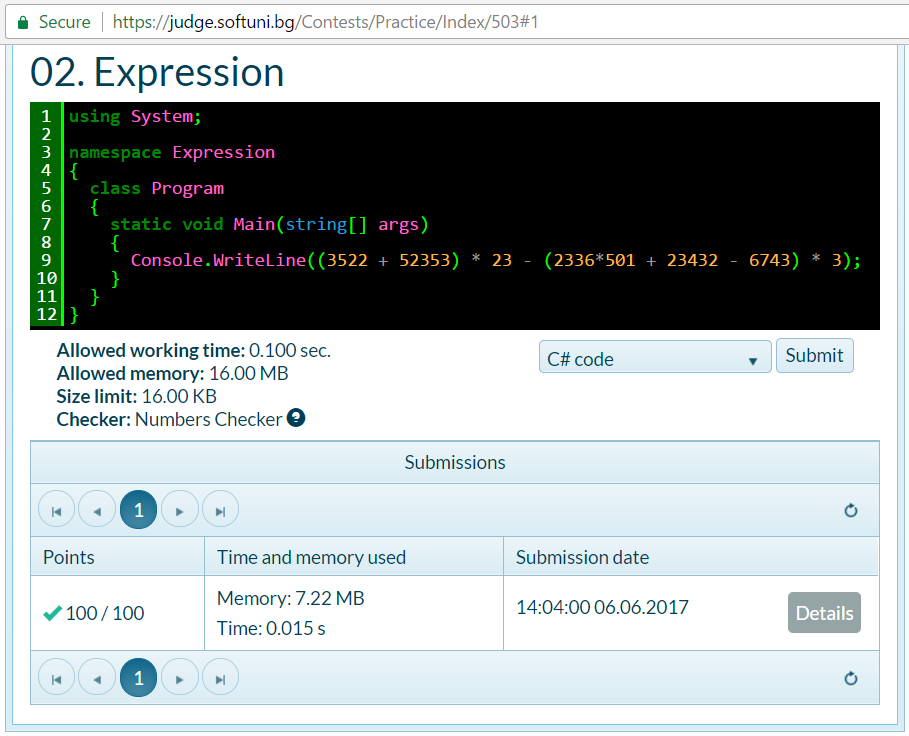# Problem: Expression

Write a console-based C# console program that calculates and prints the value of the following numerical expression:

(3522 + 52353) * 23 - (2336 * 501 + 23432 - 6743) * 3


Note: it is not allowed to previously calculate the value (for example with Windows Calculator).

## Video: Problem "Expression"

Watch a video lesson to learn how to solve the "Expression" problem step by step: https://youtu.be/JEbwS2xtbLw.

## Hints and Guidelines

Create a new C# console project with title "Expression". Find the method static void Main(string[] args) and go into its body between { and }. After that, write the code that calculates the above numerical expression and prints its value on the console. Put the above numerical expression inside the brackets of the command Console.WriteLine(…):Start the program with [Ctrl+F5] and check if the result is the same as the one in the picture:## Testing in the Judge System